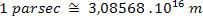Modern Physics

# measuring stars

When studying the concepts of gravitation, we saw that the average distance between the centers of the Sun and the Earth is called an astronomical unit , being represented by 1UA . We have also seen that the closest star to us (Earth) is the star Proxima Centauri . It is located at a distance of 270,000 AU. Astronomers created a unit of length to deal with these immense distances. The unit created is called a parsec . It was defined so that the distance between the center of the Earth and the center of Proxima Centaur is on the order of magnitude of 1 parsec .

The figure above shows a schematic of the process astronomers use to measure distances between stars. First they take photographs of the star at six-month intervals. Using the photos, they build the triangle like the one in the figure above. Thus, knowing the value of the base (2UA) and the value of the angles, they are able to determine the value of the distance D.

The parsec was defined as the value of the distance D when θ is equal to one second of degree: θ = 1”. As 1 degree has 60 minutes (1° = 60′) and 1 minute has 60 seconds (1 = 60”), 1 degree has 3,600 seconds (1° = 3,600”).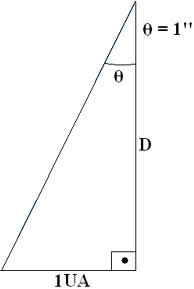In the right triangle in the figure above we have: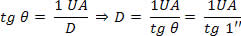Because we cannot find the value of the tangent of small angles in the usual table, we must therefore make use of a scientific calculator. To perform calculations correctly, the calculator must be in degree mode. After setting up your calculator, do the division 1/3600 and then press the tangent key. The results that are likely to appear on your calculator display will be: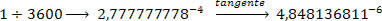This last value means that: tg 1” = 4.848136811 . 10-6.

Making some simple substitutions, we have: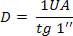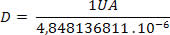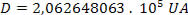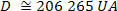As the value of 1UA corresponds to 1.49598 . 1011 m, we have: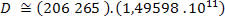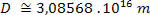In this way, we can say that: